# In theory

In the past, we thought that machines couldn’t surpass human intelligence. Things like recognizing objects with our sense of vision, was reserved for us only. In the XXI century, Artificial Intelligence puts this into question with technologies like Artificial Neural Networks.

## What is an image?

To really understand how CNNs help us classify images and recognize handwritten digits, we need to starting thinking of images in a more mathematical way. The way I see it, images are composed of 2 dimensions and 3 layers. The dimensions are length and width, whereas the layers are red, green, and blue (RGB).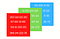If you happen to be curious about storing images in DNA, here’s an article on how I did that ;)

## CNNs overview

Classifying images of cats and dogs, or identifying handwritten digits is just the beginning. CNNs are used for face detection in some social media apps, for analysis of medical images, or even self-driving cars. A friend of mine once created a CNN to detect mitochondria!

## Convolution operation

The convolution means to take an image as an input, and pass a filter of values through it. In this case, the filter is specifying the most important features that we’re looking for in the image. The filter “scans” the image, looking for those coincidences.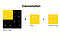1*0=0, 0*0=0, 0*1=0, 1*1=1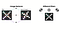Features are literally patterns or “characteristics” of an image

## Activation function

The most commonly used one is ReLU, which stands for Rectified Linear Unit. It does something called normalization, which is basically standardizing the numbers we use so there are no negative ones. If it finds a negative number, it turns it into a 0.

## Pooling

The most common type of pooling used in practice is called max pooling. It applies a 2X2 filter to the image portions that we have and literally keeps the largest value. This helps us reduce the complexity of our values without getting rid of spatial features.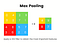4 is the largest value in the yellow quadrant, so are 9, 8, and 7 in their respective quadrants

## Classification

Interestingly, this part of the CNN process can really be done with any other algorithm that performs classification. Some examples are decision tree classifier, random forest, or logistic regression.

# In practice

Handwritten digit recognition is one of the most basic and popular AI projects for beginners. In this section, I will describe and explain the 5 steps that I followed to build that.

## #1 Import libraries

First of all, a library is a compilation of code that anyone can apply. As an analogy, we can think that there are different books in this library. We won’t find all of them useful, so we will only import some. Overall, they make things easier.

`import tensorflow as tffrom sklearn.model_selection import train_test_splitmnist = tf.keras.datasets.mnistimport matplotlib.pyplot as pltimport numpy as npfrom tensorflow.keras.models import Sequentialfrom tensorflow.keras.layers import Dense, Dropout, Activation, Flatten, Conv2D, MaxPooling2Dimport cv2import matplotlib.image as mpimg`

## #2 Prepare the data

AI is like an Olympic-level athlete: it trains to then compete. So, we need to split our resources accordingly. In this case, our data set will be split into 80% training and 20% testing.

`(x_train, y_train), (x_test, y_test) = mnist.load_data()x_train = tf.keras.utils.normalize(x_train, axis = 1)x_test = tf.keras.utils.normalize(x_test, axis = 1)IMG_SIZE = 28x_trainr = np.array(x_train).reshape(-1, IMG_SIZE, IMG_SIZE, 1)x_testr = np.array(x_test).reshape(-1, IMG_SIZE, IMG_SIZE, 1)`

## #3 Create the CNN

We will be able to see in our code how many convolution layers we have, as well as the 3 different steps that these involve: the convolution operation, the relu activation function, and max pooling.

`model = Sequential()#first convolution layermodel.add(Conv2D(64, (3, 3), input_shape = x_trainr.shape[1:]))model.add(Activation(“relu”))model.add(MaxPooling2D(pool_size=(2,2)))#second convolution layermodel.add(Conv2D(64, (3, 3)))model.add(Activation(“relu”))model.add(MaxPooling2D(pool_size=(2,2)))#third convolution layermodel.add(Conv2D(64, (3, 3)))model.add(Activation(“relu”))model.add(MaxPooling2D(pool_size=(2,2)))#classification network 1model.add(Flatten())model.add(Dense(64))model.add(Activation(“relu”))#classification network 2model.add(Dense(32))model.add(Activation(“relu”))#classification network 3model.add(Dense(10))model.add(Activation(“softmax”))model.compile(loss="sparse_categorical_crossentropy", optimizer="adam", metrics=["accuracy"])`

## #4 Train and test with existing data set

The optimizer will improve the accuracy of our model. Now, how does an Olympic-level athlete need to be? They need to be fit! So the fit method will help us train the model, after which we can make predictions with the 20% left of the data set.

`model.compile(loss="sparse_categorical_crossentropy",optimizer="adam", metrics=["accuracy"])model.fit(x_trainr, y_train, epochs = 5, validation_split = 0.3)predictions = model.predict([x_testr])`

## #5 Test it with your own numbers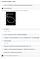5 is my favorite number 🎊

# Is AI hard?

I’ve learned that following these tutorials is incredibly easy. I’d say that you don’t even need to know python, since you could just copy and paste the code. Therefore, understanding that code is what could be challenging.

Everything around you that you call ‘life’ was made up by people who were no smarter than you — Steve Jobs

Ambitious teenager building innovative projects with Synthetic Biology and Artificial Intelligence

## More from Sofia Sanchez

Ambitious teenager building innovative projects with Synthetic Biology and Artificial Intelligence